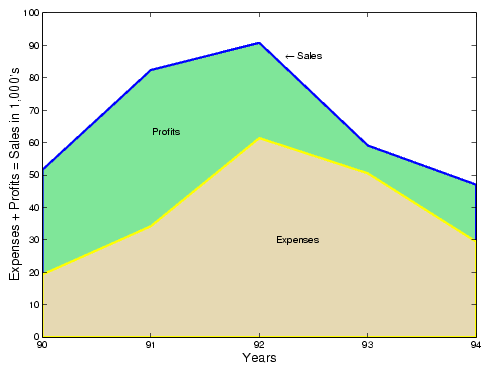GraphicsComparing Data Sets with Area Graphs

Area graphs are useful for comparing different datasets. For example, given a vector containing sales figures,

• ``````sales = [51.6 82.4 90.8 59.1 47.0];
``````

for the five-year period

• ```x = 90:94;
```

and a vector containing profits figures for the same five-year period,

• ``````profits = [19.3 34.2 61.4 50.5 29.4];
``````

display both as two separate area graphs within the same axes. Set the color of the area interior (`FaceColor`), its edges (`EdgeColor`), and the width of the edge lines (`LineWidth`). See `patch` for a complete list of properties.

• ```area(x,sales,'FaceColor',[.5 .9 .6],...
'EdgeColor','b',...
'LineWidth',2)
hold on
area(x,profits,'FaceColor',[.9 .85 .7],...
'EdgeColor','y',...
'LineWidth',2)
hold off
```

To annotate the graph, use the statements

• ```set(gca,'XTick',[90:94])
set(gca,'Layer','top')
gtext('\leftarrow Sales')
gtext('Profits')
gtext('Expenses')
xlabel('Years','FontSize',14)
ylabel('Expenses + Profits = Sales in 1,000''s','FontSize',14)```Area Graphs Pie Charts© 1994-2005 The MathWorks, Inc.